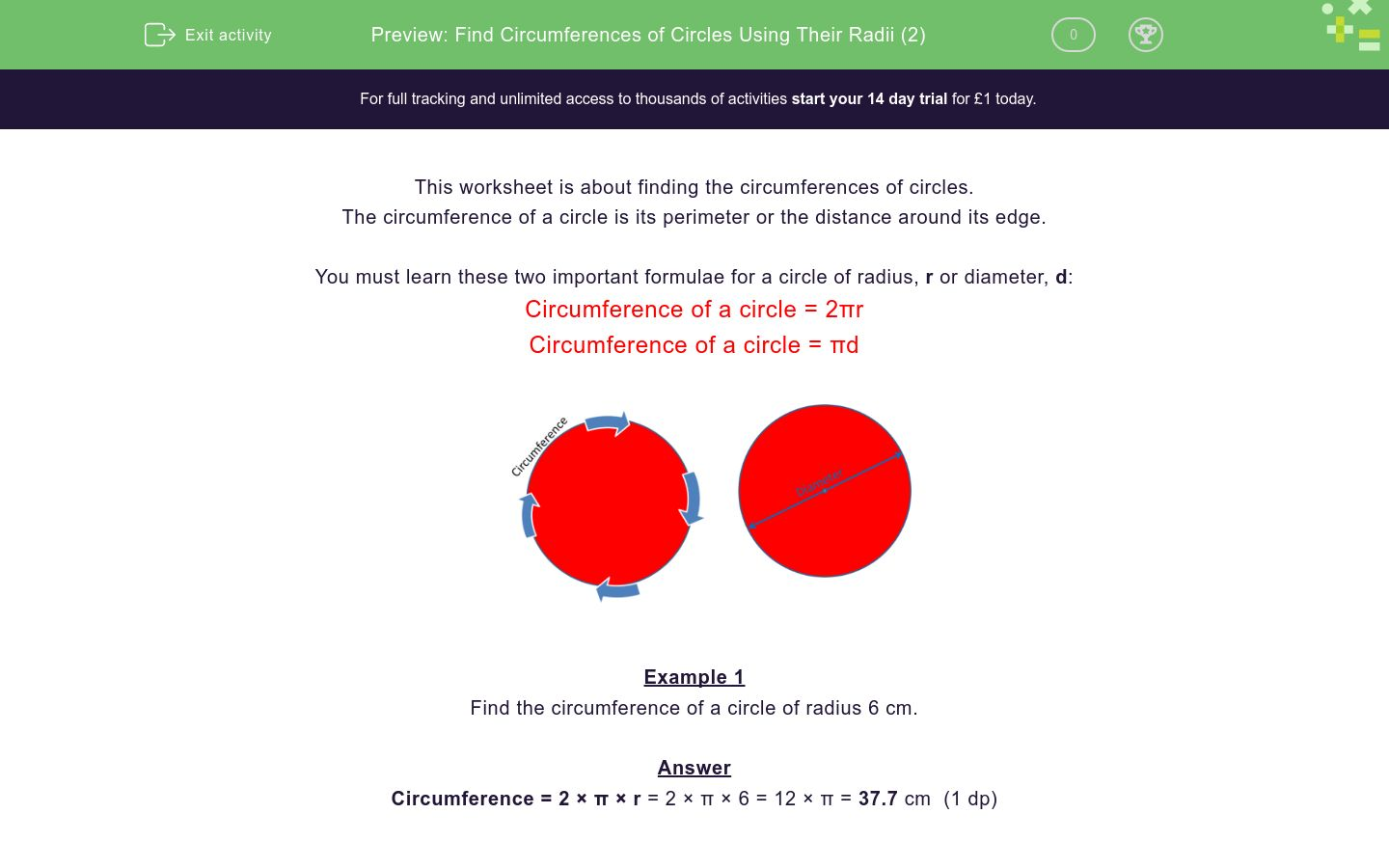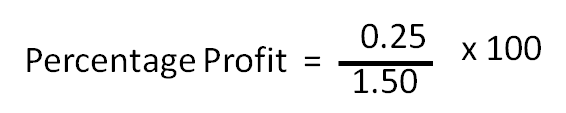# Percentage Profit and Loss

In this worksheet, students calculate percentage profits and losses.Key stage:  KS 4

Curriculum topic:  Ratio, Proportion and Rates of Change

Curriculum subtopic:  Set Up, Solve and Interpret Answers in Growth and Decay Problems

Difficulty level:### QUESTION 1 of 10

Simple profit or loss on the sale of an item is calculated by subtracting the buying price from the sale price.

Changing this into a percentage profit or loss allows you to compare sales.

Is it better to buy a pencil for 5p and sell it for 10p, or to buy a house for

£200 000 and sell it for £210 000?

The answer is debatable, however buying a pencil for 5p and selling it for 10p represents a 100% profit compared to just a 5% profit on the house.

We use these formulae to work out percentage profits or losses.Example

A bar of chocolate is bought for £1.50 and sold for £1.75.  Calculate the percentage profit.

Actual profit = 25p and starting figure = 150

or

Actual profit = £0.25 and starting figure = £1.50Percentage Profit = 16.7 % (3 s.f)

A bar of chocolate is bought for £1.20 and sold for £1.44.

Calculate the percentage profit.

(just write the number as a whole number or to 3 sig. figs. if not whole)

A bar of chocolate is bought for £1.44 and sold for £1.20.

Calculate the percentage loss.

(just write the number as a whole number or to 3 sig. figs. if not whole)

A car is bought for £1200 and sold for £1380

Calculate the percentage profit.

(just write the number as a whole number or to 3 sig. figs. if not whole)

A car is bought for £1550 and sold for £1271

Calculate the percentage loss.

(just write the number as a whole number or to 3 sig. figs. if not whole)

A book is bought for £12.50 and sold for £18

Calculate the percentage profit.

(just write the number as a whole number or to 3 sig. figs. if not whole)

A book is bought for £15 and sold for £12

Calculate the percentage loss.

(just write the number as a whole number or to 3 sig. figs. if not whole)

A lamp is bought for £156 and sold for £193.44

Calculate the percentage profit.

(just write the number as a whole number or to 3 sig. figs. if not whole)

A lamp is bought for £144 and sold for £124.

Calculate the percentage loss.

(just write the number as a whole number or to 3 sig. figs. if not whole)

A table is bought for £375 and sold for £400

Calculate the percentage profit.

(just write the number as a whole number or to 3 sig. figs. if not whole)

A table is bought for £450 and sold for £355

Calculate the percentage loss.

(just write the number as a whole number or to 3 sig. figs. if not whole)

• Question 1

A bar of chocolate is bought for £1.20 and sold for £1.44.

Calculate the percentage profit.

(just write the number as a whole number or to 3 sig. figs. if not whole)

20
EDDIE SAYS
24 ÷ 120 × 100
• Question 2

A bar of chocolate is bought for £1.44 and sold for £1.20.

Calculate the percentage loss.

(just write the number as a whole number or to 3 sig. figs. if not whole)

16.7
EDDIE SAYS
24 ÷ 144 × 100
• Question 3

A car is bought for £1200 and sold for £1380

Calculate the percentage profit.

(just write the number as a whole number or to 3 sig. figs. if not whole)

15
EDDIE SAYS
180 ÷ 1200 × 100
• Question 4

A car is bought for £1550 and sold for £1271

Calculate the percentage loss.

(just write the number as a whole number or to 3 sig. figs. if not whole)

18
EDDIE SAYS
279 ÷ 1580 × 100
• Question 5

A book is bought for £12.50 and sold for £18

Calculate the percentage profit.

(just write the number as a whole number or to 3 sig. figs. if not whole)

44
EDDIE SAYS
5.5 ÷ 12.5 × 100
• Question 6

A book is bought for £15 and sold for £12

Calculate the percentage loss.

(just write the number as a whole number or to 3 sig. figs. if not whole)

20
EDDIE SAYS
3 ÷ 15 × 100
• Question 7

A lamp is bought for £156 and sold for £193.44

Calculate the percentage profit.

(just write the number as a whole number or to 3 sig. figs. if not whole)

24
EDDIE SAYS
37.44 ÷ 156 × 100
• Question 8

A lamp is bought for £144 and sold for £124.

Calculate the percentage loss.

(just write the number as a whole number or to 3 sig. figs. if not whole)

13.9
EDDIE SAYS
20 ÷ 144 × 100
• Question 9

A table is bought for £375 and sold for £400

Calculate the percentage profit.

(just write the number as a whole number or to 3 sig. figs. if not whole)

6.67
EDDIE SAYS
25 ÷ 375 × 100
• Question 10

A table is bought for £450 and sold for £355

Calculate the percentage loss.

(just write the number as a whole number or to 3 sig. figs. if not whole)

21.1
EDDIE SAYS
95 ÷ 450 × 100
---- OR ----

Sign up for a £1 trial so you can track and measure your child's progress on this activity.

### What is EdPlace?

We're your National Curriculum aligned online education content provider helping each child succeed in English, maths and science from year 1 to GCSE. With an EdPlace account you’ll be able to track and measure progress, helping each child achieve their best. We build confidence and attainment by personalising each child’s learning at a level that suits them.